[技术干货] 分类与预测——人工神经网络

# 【Python算法】分类与预测——人工神经网络

1.人工神经网络概述
人工神经网络（Artificial Neural Networks，ANN），是一种模范动物神经网络行为特征， 进行分布式并行信息处理的算法数学模型。它以对大脑的生理研究成果为基础，依靠系统的 复杂程度，通过调整内部大量节点之间相互连接的关系，模拟大脑的某些机理与机制，从而 达到处理信息，实现一些特定的功能的目的。

2.人工神经网络具有四个基本特征
(1) 非线性：非线性关系是自然界的普遍特性，大脑的智慧就是一种非线性现象。人工 神经元处于激活或抑制两种不同的状态，这种行为在数学上表现为一种非线性关系。 具有阈值的神经元构成的网络具有更好的性能，可以提高容错性和存储容量；
(2) 非局限性：一个神经网络通常由多个神经元广泛连接而成。一个系统的整体行为不 仅取决于单个神经元的特征，而且可能主要由单元之间的相互作用、相互连接所决 定。通过单元之间的大量连接模拟大脑的非局限性。联想记忆就是非局限性的典型 例子；
(3) 非常定性：人工神经网络具有自适应、自组织、自学习能力。神经网络不但处理的 信息可以有各种变化，而且在处理信息的同时，非线性动力系统本身也在不断变化。 经常采用迭代过程描写动力系统；
(4) 非凸性：一个系统的演化方向，在一定条件下将取决于某个特定的状态函数。例如 能量函数，它的极值相应用于系统比较稳定的状态。非凸性是指这种函数有多个极 值，故系统具有多个较稳定的平衡态，这将导致系统演化的多样性。

3.神经元（节点）组成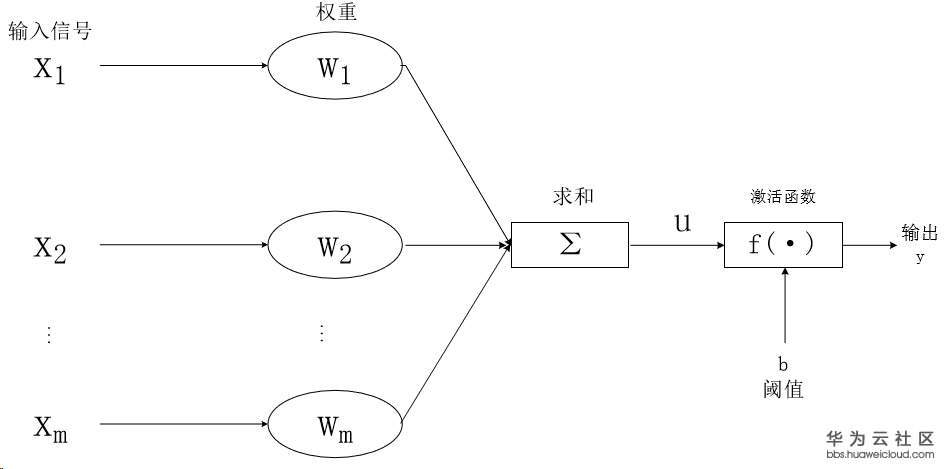一个神经元由输入值，激活函数，输出值，权重组成，输入值经过激活函数变为输出值，再与权重相乘变为下一个神经元的输入值。由这样的单位组成的网络就是神经网络，
在实际生活中我们给更多的使用BP神经网络，即反向传播神经网络。

4.BP神经网络的建模步骤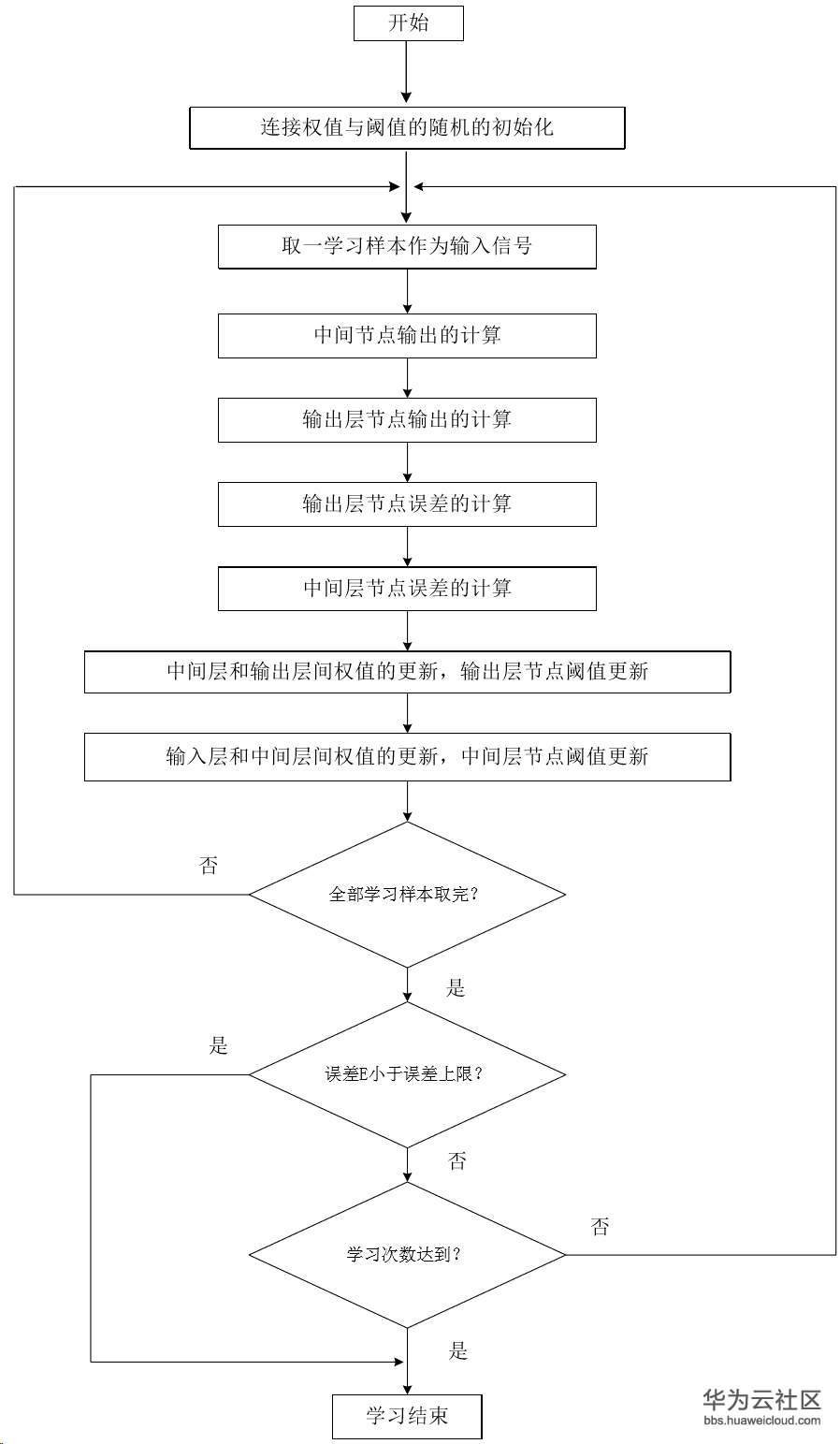在反向传播算法中我们涉及到了求梯度的问题，但是求导运算对于计算机来说计算机太过复杂，于是诞生了牛顿法、拟牛顿法、梯度下降法等方式来帮助电脑计算梯度，在实际生活中我们常用

5.梯度下降法
梯度类似于微分，微分是针对单变量，而梯度是针对向量。
因为直接获得最优解的计算量过大，所以通过均方误差的分析，采用迭代的方式来一步一步获得局部最优解，这就是梯度下降法。梯度下降法通过电脑擅长的方式——迭代，极大的减少了求梯度的计算量，但是对于一些较为复杂的函数而言，梯度下降法获得的更多是局部最优解而不是全局最优解。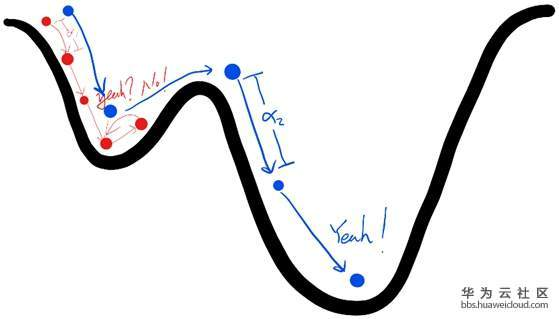由此可见，使局部最优解接近全局最优解的方式便是调节学习率。
红点和蓝点实际上就代表了反向传播算法，下山的路径其实就代表着算法中一直在寻找的参数最优解，山上当前点的最陡峭的方向实际上就是代价函数在这一点的梯度方向，场景中观测最陡峭方向所用的工具就是微分。在下一步要走多长就是由我们算法中的学习率α所定义。
梯度下降法本身具有弹性，可以容忍不完善的数据，如果我们不能完美地描述函数，或者我们意外的走错了一步，都不至于前功尽弃。

其中误差计算通常为均方误差，即（目标值 – 实际值）的平方，多采用均方误差的原因有以下几点：

1. 使用误差的平方，我们可以很容易使用代数计算出梯度下降的斜率。
2. 误差函数平滑且连续，这使梯度下降法很好的发挥了作用——没有发生间断，也没有发生跳跃。
3. 越接近最小值，梯度就越小，这意味着，如果我们使用这个函数调节步长，超调的风险就会变得较小。
从结构上讲，BP网络具有输入层、隐藏层和输出层；从本质上讲，BP算法就是以均方误差为目标函数、采用梯度下降法来计算目标函数的最小值。
设第i层和第j层之间的权重为w(i,j)，对当前层权重反向传播修正权重的公式为：

Δw(i,j) = α ····· 误差 · output(j) · (1 - output(j)) · output(i)

通过反向传播修正链上的权重来改善输出情况。

6.操作系统

操作机：Linux_Ubuntu
操作机默认用户：root

7.实验工具
7.1.python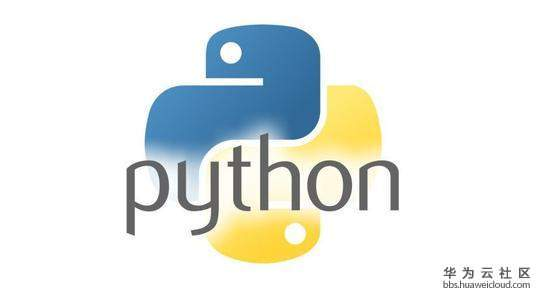Python是一种计算机程序设计语言。是一种动态的、面向对象的脚本语言，最初被设计用于编写自动化脚本(shell)，随着版本的不断更新和语言新功能的添加，越来越多被用于独立的、大型项目的开发。Python已经成为最受欢迎的程序设计语言之一。自从2004年以后，python的使用率呈线性增长。2011年1月，它被TIOBE编程语言排行榜评为2010年度语言。
由于Python语言的简洁性、易读性以及可扩展性，在国外用Python做科学计算的研究机构日益增多，一些知名大学已经采用Python来教授程序设计课程。例如卡耐基梅隆大学的编程基础、麻省理工学院的计算机科学及编程导论就使用Python语言讲授。
众多开源的科学计算软件包都提供了Python的调用接口，例如著名的计算机视觉库OpenCV、三维可视化库VTK、医学图像处理库ITK。而Python专用的科学计算扩展库就更多了，例如如下3个十分经典的科学计算扩展库：NumPy、SciPy和matplotlib，它们分别为Python提供了快速数组处理、数值运算以及绘图功能。因此Python语言及其众多的扩展库所构成的开发环境十分适合工程技术、科研人员处理实验数据、制作图表，甚至开发科学计算应用程序。

7.2.NumPy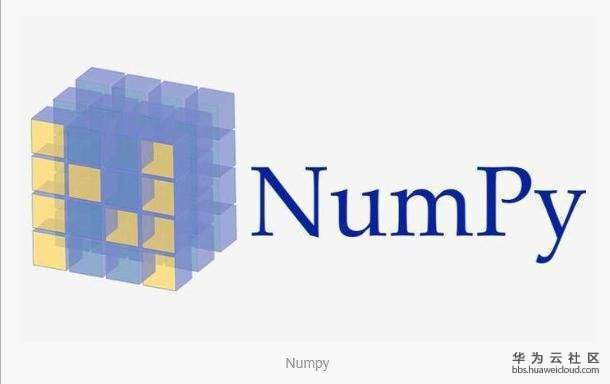NumPy系统是Python的一种开源的数值计算扩展。这种工具可用来存储和处理大型矩阵，比Python自身的嵌套列表（nested list structure)结构要高效的多（该结构也可以用来表示矩阵（matrix））。
NumPy（Numeric Python）提供了许多高级的数值编程工具，如：矩阵数据类型、矢量处理，以及精密的运算库。专为进行严格的数字处理而产生。多为很多大型金融公司使用，以及核心的科学计算组织如：Lawrence Livermore，NASA用其处理一些本来使用C++，Fortran或Matlab等所做的任务。

7.3.SciPySciPy是一款方便、易于使用、专为科学和工程设计的Python工具包.它包括统计,优化,整合,线性代数模块,傅里叶变换,信号和图像处理,常微分方程求解器等等.

8.导入numpy，scipy.special

实验中将使用人工神经网络对含有110个手写识别数据的数据集进行识别。

```#导入库
import numpy
#numpy是高级的数学计算库
import scipy.special
#在这里用到scipy的expit（）函数作为sigmod（）函数
import matplotlib.pyplot```

9.学习人工神经网络类的框架

#### 人工神经网络类的整体框架如下：

```class neuralNetwork:
def __init__(self, inputnodes, hiddennodes, outputnodes, learningrate):
pass

def train(self, inputs_list, targets_list):
pass

def query(self, inputs_list):```

• self.inodes表示输入节点

• self.hnodes表示隐层节点

• self.onodes表示输出节点

• self.wih表示输入层到隐层的权重

• self.who表示隐层到输出层的权重

• self.lr表示学习率

• self.activation表示激活函数

编写_init_函数，完整代码如下：

```class neuralNetwork:
def __init__(self, inputnodes, hiddennodes, outputnodes, learningrate):

self.inodes = inputnodes   #输入节点
self.hnodes = hiddennodes   #隐层节点
self.onodes = outputnodes   #输出节点

#权重链接
self.wih = numpy.random.normal(0.0, pow(self.inodes, -0.5), (self.hnodes, self.inodes))
self.who = numpy.random.normal(0.0, pow(self.hnodes, -0.5), (self.onodes, self.hnodes))

# 学习率
self.lr = learningrate

# 激活函数直接调用self.activation_function
self.activation_function = lambda x: scipy.special.expit(x)

pass```

10.train函数实现整个神经网络的训练过程

首先实现正向传播，隐层节点的输入值等于输入层的输入值乘以wih权重，代码如下：

```        hidden_inputs = numpy.dot(self.wih, inputs)
hidden_outputs = self.activation_function(hidden_inputs)```

输出层的输入值等于隐层输出值乘以who权重 。代码如下：

```        final_inputs = numpy.dot(self.who, hidden_outputs)
final_outputs = self.activation_function(final_inputs)```

正向传播结束后，使用反向传播算法调节链上权重。

```output_errors = targets - final_outputs
hidden_errors = numpy.dot(self.who.T, output_errors)

self.who += self.lr * numpy.dot((output_errors * final_outputs * (1.0 - final_outputs)), numpy.transpose(hidden_outputs))
self.wih += self.lr * numpy.dot((hidden_errors * hidden_outputs * (1.0 - hidden_outputs)), numpy.transpose(inputs))```

train函数完整代码如下：

``` def train(self, inputs_list, targets_list):
# 将输入和目标值转换为矩阵（numpy的数组）
inputs = numpy.array(inputs_list, ndmin=2).T
targets = numpy.array(targets_list, ndmin=2).T

hidden_inputs = numpy.dot(self.wih, inputs)
hidden_outputs = self.activation_function(hidden_inputs)

final_inputs = numpy.dot(self.who, hidden_outputs)
final_outputs = self.activation_function(final_inputs)

output_errors = targets - final_outputs
hidden_errors = numpy.dot(self.who.T, output_errors)

self.who += self.lr * numpy.dot((output_errors * final_outputs * (1.0 - final_outputs)), numpy.transpose(hidden_outputs))
self.wih += self.lr * numpy.dot((hidden_errors * hidden_outputs * (1.0 - hidden_outputs)), numpy.transpose(inputs))

pass```

11.query函数

实现输入测试数据，对得到的结果进行评估。将输入值经过正向传播算法得到结果即可
代码如下：

``` def query(self, inputs_list):
#将输入转换为矩阵
inputs = numpy.array(inputs_list, ndmin=2).T

hidden_inputs = numpy.dot(self.wih, inputs)
hidden_outputs = self.activation_function(hidden_inputs)

final_inputs = numpy.dot(self.who, hidden_outputs)
final_outputs = self.activation_function(final_inputs)

return final_outputs```

注意：将以上函数都写入在框架内。

12.设定参数

Mnist手写数据图片为28x28的分辨率，所以输入层采用784(28*28)个节点，隐层采用200(可自行调整)个节点，输出层采用10(0~9)个节点，学习率为0.1
代码如下：

```# 输入层节点 隐层节点 输出层节点
input_nodes = 784
hidden_nodes = 200
output_nodes = 10

#学习率设定为0.1
learning_rate = 0.1

#创建出训练的神经网络
n = neuralNetwork(input_nodes,hidden_nodes,output_nodes, learning_rate)```

13.读取训练数据集

代码如下：

```# 打开mnist_CSV训练文件
training_data_file = open("/mnt/dataset_54/mnist_train_100_bcdb280d7666ea5865e56b56d47167ec.csv", 'r')
training_data_list = training_data_file.readlines()
training_data_file.close()```

14.用训练数据集对神经网络进行训练

代码如下：

```#训练网络

# epochs表示训练世代
epochs = 5

for e in range(epochs):
for record in training_data_list:
# 去掉，
all_values = record.split(',')
# inputs进行转换
inputs = (numpy.asfarray(all_values[1:]) / 255.0 * 0.99) + 0.01
# 把所有0转换为0.01
targets = numpy.zeros(output_nodes) + 0.01
# 把所有1转换为0.99
targets[int(all_values)] = 0.99
n.train(inputs, targets)
pass
pass```

15.读取测试数据集

代码如下：

```# 打开mnist_CSV测试文件
test_data_file = open("/mnt/dataset_54/mnist_test_10_de377bdbad1fe5920e467c5dee8cb718.csv", 'r')
test_data_list = test_data_file.readlines()
test_data_file.close()```

16.将测试数据集读入训练好的神经网络中并输出结果

代码如下：

```# 测试神经网络

# 用scorecaed统计正确的数目
scorecard = []

for record in test_data_list:
# 去除测试数据中的逗号
all_values = record.split(',')
# 正确的答案放在第零位标签
correct_label = int(all_values)
print(correct_label,"correct answer")

# 处理测试数据

inputs = (numpy.asfarray(all_values[1:]) / 255.0 * 0.99) + 0.01
outputs = n.query(inputs)

#获得输出的概率的最大值所对应的标签
label = numpy.argmax(outputs)

print(outputs)
print(label,"network's answer")
print("\n")

#计量正确识别数
if (label == correct_label):
#正确的就在scorecard加个1
scorecard.append(1)
else:
# 错误的就在scorecard加个0
scorecard.append(0)
pass

pass```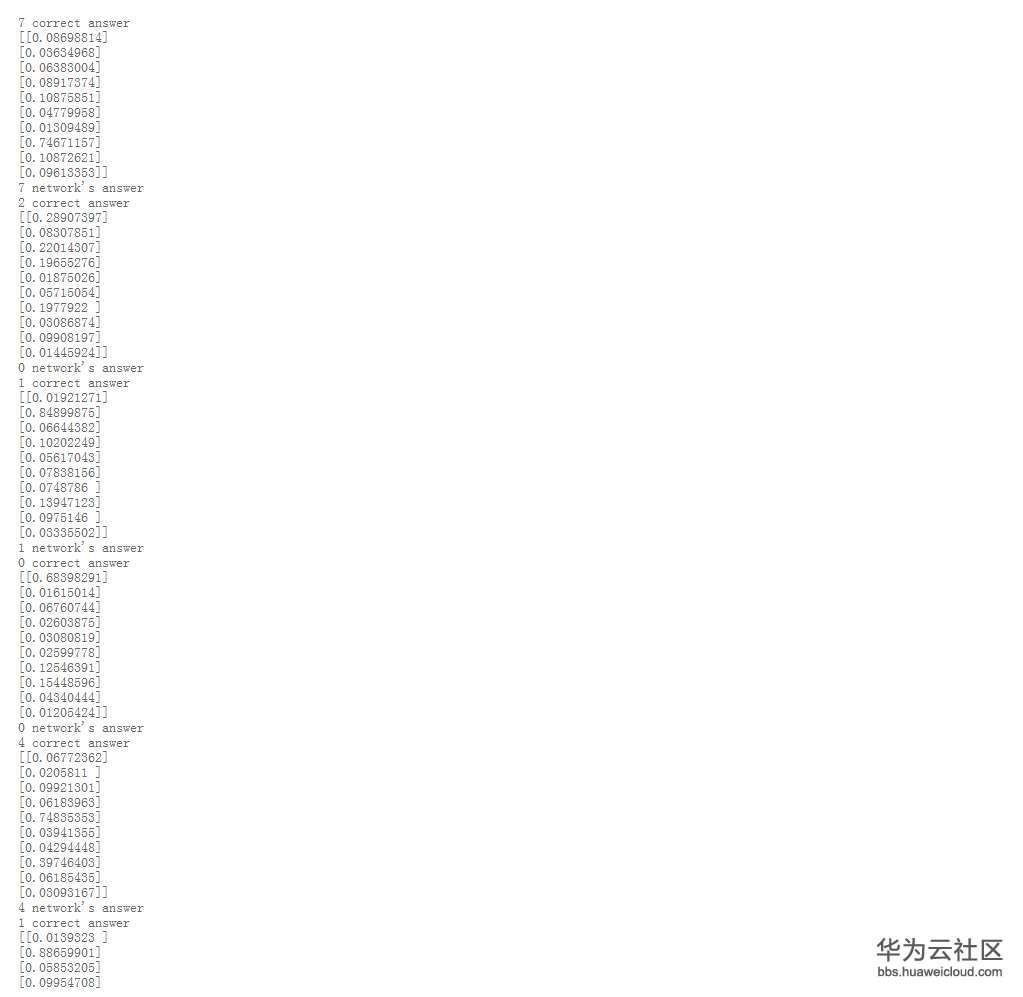这是其中三组输出结果，correct answer是图片的标签即正确答案，network's answer是神经网络识别的结果即测试结果。中间的矩阵是神经网络的十个输出节点输出的值，自上而下分别代表着0~9的概率，概率高者即为神经网络得到的答案。

```#导入库
import numpy
#numpy是高级的数学计算库
import scipy.special
#在这里用到scipy的expit（）函数作为sigmod（）函数
import matplotlib.pyplot

class neuralNetwork:

def __init__(self, inputnodes, hiddennodes, outputnodes, learningrate):

self.inodes = inputnodes   #输入节点
self.hnodes = hiddennodes   #隐层节点
self.onodes = outputnodes   #输出节点

#权重链接
self.wih = numpy.random.normal(0.0, pow(self.inodes, -0.5), (self.hnodes, self.inodes))
self.who = numpy.random.normal(0.0, pow(self.hnodes, -0.5), (self.onodes, self.hnodes))
# 学习率
self.lr = learningrate

# 激活函数直接调用self.activation_function
self.activation_function = lambda x: scipy.special.expit(x)

pass

def train(self, inputs_list, targets_list):
# 将输入和目标值转换为矩阵（numpy的数组）
inputs = numpy.array(inputs_list, ndmin=2).T
targets = numpy.array(targets_list, ndmin=2).T

hidden_inputs = numpy.dot(self.wih, inputs)
hidden_outputs = self.activation_function(hidden_inputs)

final_inputs = numpy.dot(self.who, hidden_outputs)
final_outputs = self.activation_function(final_inputs)

output_errors = targets - final_outputs
hidden_errors = numpy.dot(self.who.T, output_errors)

self.who += self.lr * numpy.dot((output_errors * final_outputs * (1.0 - final_outputs)), numpy.transpose(hidden_outputs))
self.wih += self.lr * numpy.dot((hidden_errors * hidden_outputs * (1.0 - hidden_outputs)), numpy.transpose(inputs))

pass

def query(self, inputs_list):
#将输入转换为矩阵
inputs = numpy.array(inputs_list, ndmin=2).T

hidden_inputs = numpy.dot(self.wih, inputs)
hidden_outputs = self.activation_function(hidden_inputs)

final_inputs = numpy.dot(self.who, hidden_outputs)
final_outputs = self.activation_function(final_inputs)

return final_outputs

# 输入层节点 隐层节点 输出层节点
input_nodes = 784
hidden_nodes = 200
output_nodes = 10

#学习率设定为0.1
learning_rate = 0.1

#创建出训练的神经网络
n = neuralNetwork(input_nodes,hidden_nodes,output_nodes, learning_rate)

# 打开mnist_CSV训练文件
training_data_file = open("/mnt/dataset_54/mnist_train_100_bcdb280d7666ea5865e56b56d47167ec.csv", 'r')
training_data_list = training_data_file.readlines()
training_data_file.close()

#训练网络

# epochs表示训练世代
epochs = 5

for e in range(epochs):
for record in training_data_list:
# 去掉，
all_values = record.split(',')
# inputs进行转换
inputs = (numpy.asfarray(all_values[1:]) / 255.0 * 0.99) + 0.01
# 把所有0转换为0.01
targets = numpy.zeros(output_nodes) + 0.01
# 把所有1转换为0.99
targets[int(all_values)] = 0.99
n.train(inputs, targets)
pass
pass

# 打开mnist_CSV测试文件
test_data_file = open("/mnt/dataset_54/mnist_test_10_de377bdbad1fe5920e467c5dee8cb718.csv", 'r')
test_data_list = test_data_file.readlines()
test_data_file.close()

# 测试神经网络

# 用scorecaed统计正确的数目
scorecard = []

for record in test_data_list:
# 去除测试数据中的逗号
all_values = record.split(',')
# 正确的答案放在第零位标签
correct_label = int(all_values)
print(correct_label,"correct answer")
# 处理测试数据
inputs = (numpy.asfarray(all_values[1:]) / 255.0 * 0.99) + 0.01
outputs = n.query(inputs)
#获得输出的概率的最大值所对应的标签
label = numpy.argmax(outputs)
print(outputs)
print(label,"network's answer")
#计量正确识别数
if (label == correct_label):
#正确的就在scorecard加个1
scorecard.append(1)
else:
# 错误的就在scorecard加个0
scorecard.append(0)
pass

pass

# 计算正确率
scorecard_array = numpy.asarray(scorecard)
print ("performance = ", scorecard_array.sum() / scorecard_array.size)```